### Home > INT3 > Chapter Ch10 > Lesson 10.3.3 > Problem10-185

10-185.
1. Consider that ln(2) ≈ 0.69315 and ln(3) ≈ 1.0986. Homework Help ✎

1. Why is ln(2) < 1 and ln(3) > 1? For what value of x does ln(x) = 1?2. Use the properties of logarithms to write each value below as an expression involving ln(2) and/or ln (3). Then use the given values for ln(2) and ln(3) to evaluate each of the expressions without a calculator.

 i. ln(6) ii. ln(12) iii. ln(16) iv. ln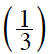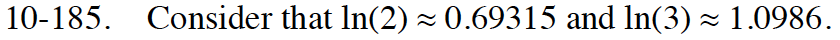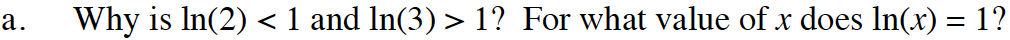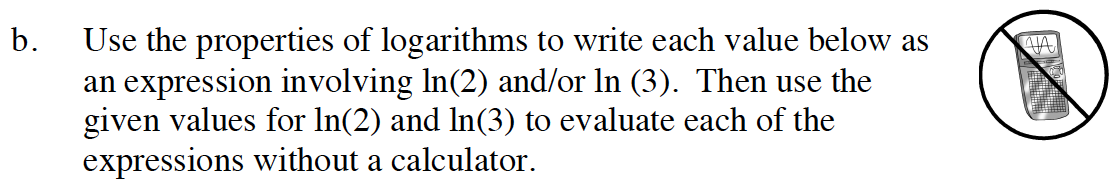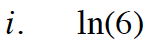ln6 = ln(2 · 3)

ln6 = ln2 + ln3

ln6 = (0.69315) + (1.0986)
ln6 = 1.79175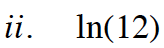See part (a).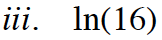See part (a).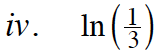$\ln\frac{1}{3} = \ln\left(3^{-1}\right)$

$\ln\frac{1}{3} = -\ln3$